ISSAC 96 - Hypergeometric Function Representations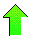Contents News View

 2F1 Example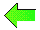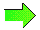Let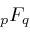denote the restriction of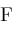to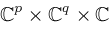.  Then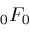,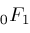, and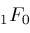are representable by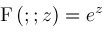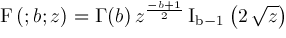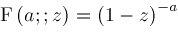presenting no further challenge to us.

We proceed to a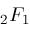example which is more interesting.  Let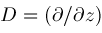be the operator for differentiation.  The following shift relations are known: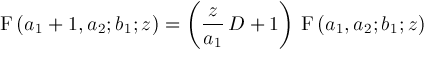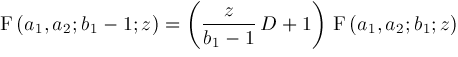Also, the following contiguity relations are known: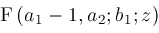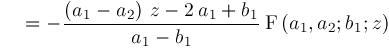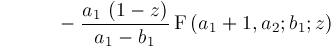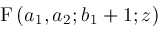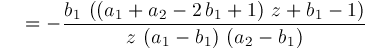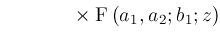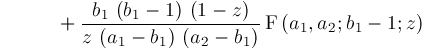Using these shift and contiguity relations, we can start from almost any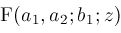representation to obtain any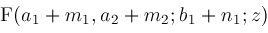representation where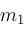,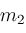,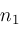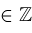.  The denominators appearing in the shift relations and contiguity relations are troublesome since we can't let them become zero.

We show how to compute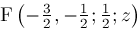by starting from the known formula for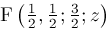Known: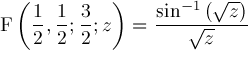Shift: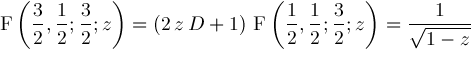Contiguity: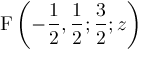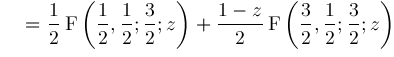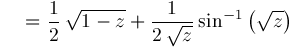Contiguity: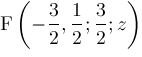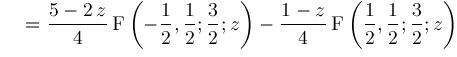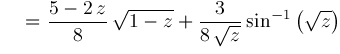Shift: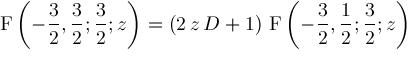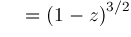Contiguity: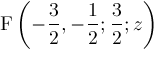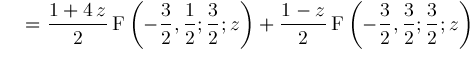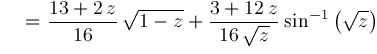Shift: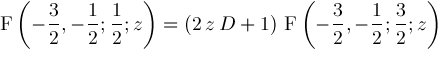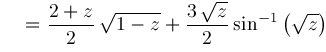Hence, we conclude: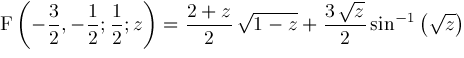This formula is not explicitly listed in .  Neither Mathematica 2.2 nor Maple 5.3 will compute it.  Macsyma 419.0 does compute it, but returns a wrong answer.

We will develop a strategy using shift relations and contiguity relations forwhere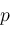and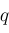are arbitrary (subject to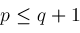) to compute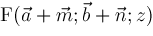from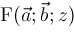.  First, we will study shift relations and contiguity relations for general©2004-2018 Planet Quantum Kelly Roach